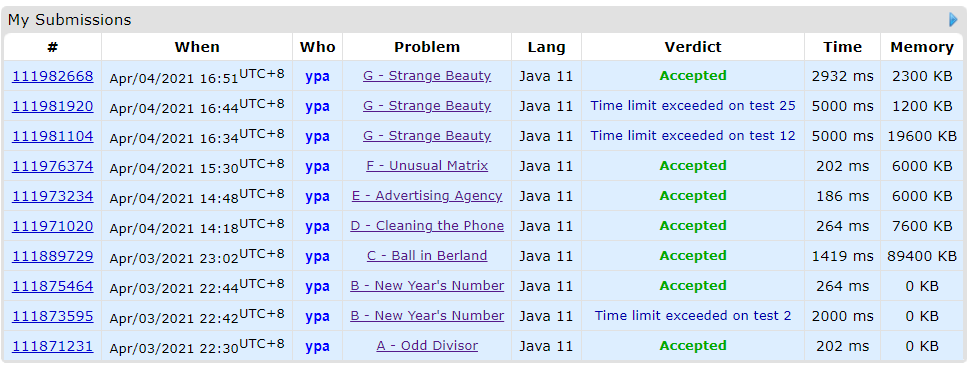This round of Div3 is interesting. Problem G is a basic DP + number theory + very strict time limit. The problem F is a complex implementation problem. The problem D sounds like DP. But actually just a greedy and binary search problem. Interesting.The time limit for problem G is still a bit interesting

## G. Strange Beauty

### Solution

Can be a DP problem.  This question has a very strict time limit.

Conclusion first: $dp_i=\max{(dp_i, dp_{pos_{factor}} + count_{a_i})}$. $pos_{factor}$ represents the position of the $factor$ of $a_i$. $count_{a_i}$ represents the current number of occurrences of this $a_i$.

Now we move to the details:

First of all, sort the $a$. And collect all the repeated $a_i$ into the $count$. After that, record the position of $a_i$ into $pos_{a_i}$.

For any ${1}\leq{a_i}\leq{2\cdot{10^5}}$. the number of factor does not exceed 1000 ($500^2=250000$). So for $dp_i$, just find out this 1000 factors by brute force search.

For TLE:

$Pos$ should use array directly. Because ${1}\leq{a_i}\leq{2\cdot{10^5}}$, and use Map will spend one more $log(n)$ time.

Also the $count$ is very important. Otherwise it only +1 in the dp. It's super slow.

## F. Unusual Matrix

### Solution

Think about the first row. After we decided if XOR this row or not. The result is also determined.

Because the XOR for column is determined by first row. So just need scan each row, and it's very clear if it need XOR by row or not.

### Solution

Count the number of occurrences of each number. Then choose from big to small. When it is found that there are more than $k$ from a certain number, count the number of combinations.

## D. Cleaning the Phone

### Solution

Greedy and binary search.

The points of application are only two different value. So for the application with same points, release applications that take up more memory firstly.

So just enumerate the number of 1 point application which is released. Then binary search the number of 2 point application.

Be careful. This process need calculate prefix sum. And the maximum value of $a_i$ is $10^9$. So int is not enough.

## C. Ball in Berland

### Solution

Enumerate the first pair. Then calculate other possibilities through set operations.

The thing is, the problem doesn't guarantee that the entered pairing is not repeated. For example $a=1,\ b=1$, at same time, $a=1,\ b=1$. So need use Set to eliminate duplication.

## B. New Year's Number

### Solution

The maximum value of $n$ is $10^6$. So just brute force. Enumerate the number of 2020 from 0 to 500. After that, see if it can be divisible by 2021.

Don't enumerate 2020 and 2021 at same time. Otherwise you will get TLE.

## A. Odd Divisor

### Solution

Keep dividing by 2 until it is not divisible by 2, and see what is left.

### Code蜀ICP备19018968号